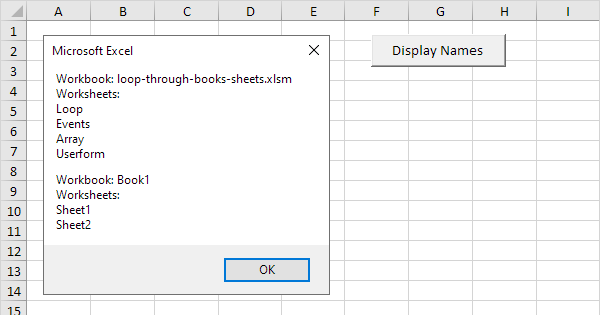# How to Use Loop through Books and Sheets in Excel

Oct 19, 2020 • edited Oct 21, 2020Below we will look at a program in Excel VBA that loops through all open workbooks and worksheets, and displays all the names.

Situation:Add the following code lines to the command button:

1. First, we declare two objects and one variable. One object of type Workbook we call book, one object of type Worksheet we call sheet, and a variable of type String we call text.

``````          Dim book As Workbook, sheet As Worksheet, text As String
``````

2. We want to loop through all open workbooks. To achieve this, add the following code line:

``````          For Each book In Workbooks
``````

3. We write the text "Workbook: ", the name of the workbook, and the text "Worksheets: "" to the variable text.

``````          text = text &amp; &quot;Workbook: &quot; &amp; book.Name &amp; vbNewLine &amp; &quot;Worksheets: &quot; &amp; vbNewLine
``````

Note: you can use the & operator to concatenate (join) elements. To start a new line, you can use vbNewLine.

4. To loop through all the worksheets of a workbook, add the following code line:

``````          For Each sheet In book.Worksheets
``````

5. We write the names of the worksheets of a workbook to the variable text.

``````          text = text &amp; sheet.Name &amp; vbNewLine
``````

6. Close the second loop.

``````          Next sheet
``````

``````          text = text &amp; vbNewLine
``````

8. Don't forget to close the first loop.

``````          Next book
``````

9. Finally, we display the variable text using a MsgBox.

``````          MsgBox text
``````

10. Test the program. Before you click on the command button, give your worksheets some descriptive names and open a blank workbook with three worksheets.

Result:#Tutorial#How To#VBA#Workbook#Worksheet

How To Use Excel VBA For Loop through Entire Column

How To Use Macro Comments In Excel's Vba